The REG Procedure

Computer Resources in Regression Analysis

The REG procedure is efficient for ordinary regression; however, requests for optional features can greatly increase the amount of time required.

The major computational expense in the regression analysis is the collection of the crossproducts matrix. For p variables and n observations, the time required is proportional to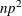. For each model run, PROC REG needs time roughly proportional to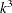, where k is the number of regressors in the model. Include an additional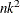for the R, CLM, or CLI option and anotherfor the INFLUENCE option.

Most of the memory that PROC REG needs to solve large problems is used for crossproducts matrices. PROC REG requires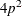bytes for the main crossproducts matrix plus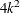bytes for the largest model. If several output data sets are requested, memory is also needed for buffers.

See the section Input Data Sets for information about how to use TYPE=SSCP data sets to reduce computing time.